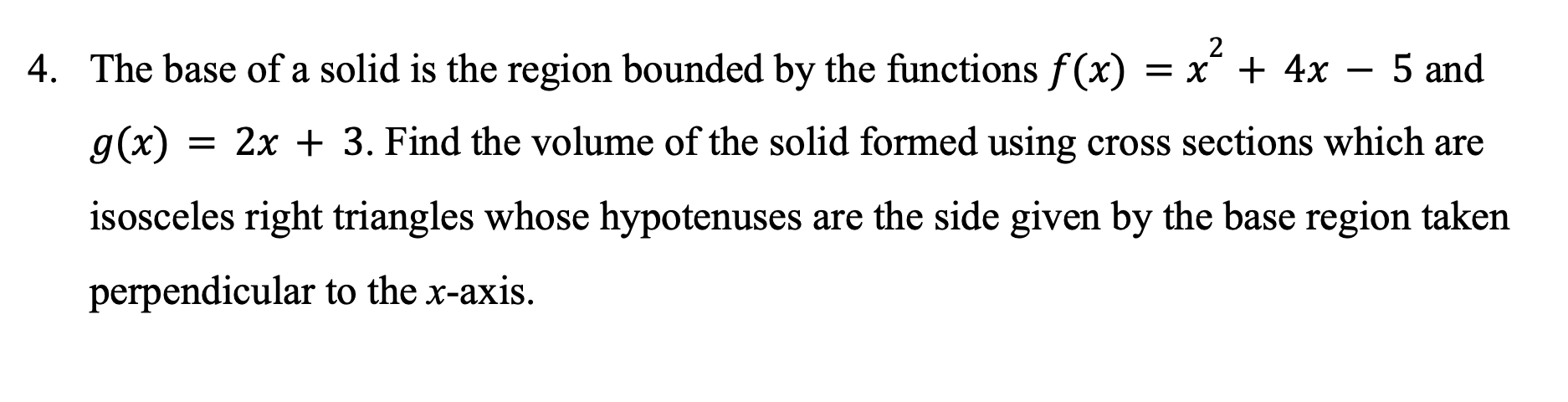# Question 4. The base of a solid is the region bounded by the functions f(x) = x² + 4x – 5 and + g(x) 2x + 3. Find the volume of the solid formed using cross sections which are isosceles right triangles whose hypotenuses are the side given by the base region taken perpendicular to the x-axis.